Ministério da Ciência, Tecnologia, Inovações e Comunicações CBPFIndex CENTRO BRASILEIRO DE PESQUISAS FÍSICAS17/07/2019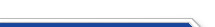Usuário: Senha: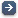Crie sua conta aqui · Busca rápida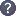Procurar por: Publicações Projetos EventosIr para a busca avançada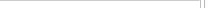Notas de Física
 ${\mathbb Z}_2\times {\mathbb Z}_2$-graded Lie Symmetries of the Lévy-Leblond Equations Data do cadastro: 29/09/2016 Publicação/Divulgação: 29/09/2016 Resp. pelo cadastro: Status atual: Publicado Ano da publicação: 2016 Descrição: The first-order differential L\'evy-Leblond equations (LLE's) are the non-relativistic analogs of the Dirac equation, being square roots of ($1+d$)-dimensional Schr\"odinger or heat equations. Just like the Dirac equation, the LLE's possess a natural supersymmetry. In previous works it was shown that non supersymmetric PDE's (notably, the Schr\"odinger equations for free particles or in the presence of a harmonic potential), admit a natural ${\mathbb Z}_2$-graded Lie symmetry.\par In this paper we show that, for a certain class of supersymmetric PDE's, a natural ${\mathbb Z}_2\times{\mathbb Z}_2$-graded Lie symmetry appears. In particular, we exhaustively investigate the symmetries of the $(1+1)$-dimensional L\'evy-Leblond Equations, both in the free case and for the harmonic potential. In the free case a ${\mathbb Z}_2\times{\mathbb Z}_2$-graded Lie superalgebra, realized by first and second-order differential symmetry operators, is found. In the presence of a non-vanishing quadratic potential, the Schr\"odinger invariance is maintained, while the ${\mathbb Z}_2$- and ${\mathbb Z}_2\times{\mathbb Z}_2$- graded extensions are no longer allowed. \par The construction of the ${\mathbb Z}_2\times {\mathbb Z}_2$-graded Lie symmetry of the ($1+2$)-dimensional free heat LLE introduces a new feature, explaining the existence of first-order differential symmetry operators not entering the super Schr\"odinger algebra. Número: CBPF-NF-004/16 Autores: F. Toppan; Z. Kuznetsova; N. Aizawa; H. Tanaka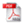Download do PDF (535 KB)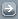Todos EventosTodos PublicaçõesTodasArtigos de Div. CientíficaArtigos em Revistas Nac.Artigos em Revistas Int.Artigos em Eventos Nac.Artigos em Eventos Int.Livros InteirosCapítulos de LivroPropriedades IntelectuaisÁudioPatentesProgramas de computadorModelos de utilidadeMarcasOutras prop. intel.TecnologiasKnow-howServiços tecnológicosTecnologias inovadorasTeses de DoutoradoDissertações de MestradoProjetos de GraduaçãoCiência e SociedadeDocumentos HistóricosMonografiasNotas de FísicaNotas TécnicasOutras Produções
 · Principal    |    CBPF    |    · Copyright 2004 - CBPF. Rio de Janeiro/BrasilCentro Brasileiro de Pesquisas Físicasv 1.1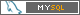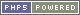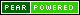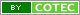Pág. gerada em 0.01 s Total de consultas SQL: 33